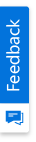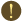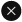We use cookies to give you the best experience on our website. If you continue to browse, then you agree to our privacy policy and cookie policy.Unfortunately, activation email could not send to your email. Please try again.# List of Available Diagram Drawing Tools

Platform: WinForms |
Control: Diagram

Drawing tool allows you to draw any kind of node/connector during runtime by clicking and dragging on the Diagram page.

## Shapes Tools

There are multiple tool contains in diagram drawing tools to draw a shapes likes RectangleBased tools, PolyLine Based tools and Line based tools.

## Rectangle Based Tools

Initially need to select a particular tool from the toolbar and after selecting simply draw it in the diagram will create the respective node as per the tool selected.

## Rectangle Tool

It is used to draw the Rectangle shape in run time. The following code illustrates how to activate the rectangle tool.

[C#]

```// Activates the Rectangle tool.
diagram1.Controller.ActivateTool("RectangleTool");
```

[VB]

```' Activates the rectangle tool.
diagram1.Controller.ActivateTool("RectangleTool ");
```

## RoundRectangle Tool

It is used to draw the rectangle with rounded corners in run time. The following code illustrates how to activate the round rectangle tool.

[C#]

```// Activates the round rectangle tool.
diagram1.Controller.ActivateTool("RoundRectTool");
```

[VB]

```' Activates the round rectangle tool.
diagram1.Controller.ActivateTool("RoundRectTool ");
```

## EllipseTool

It will help us to draw an ellipse shape in runtime. The following code illustrates how to activate the ellipse node tool.

[C#]

```// Activates the ellipse tool.
diagram1.Controller.ActivateTool("EllipseTool");
```

[VB]

```' Activates the ellipse tool.
diagram1.Controller.ActivateTool("EllipseTool")
```

## SemiCircleTool

It is used to draw the half of the ellipse in runtime. The following code illustrates how to activate the semicircle tool.

[C#]

```' Activates the semicircle tool.
diagram1.Controller.ActivateTool("SemiCircleTool");
```

[VB]

```' Activates the semicircle tool.
diagram1.Controller.ActivateTool("SemiCircleTool");
```

## BitmapTool

It is used to draw the bitmap or image node in runtime. The following code illustrates how to activate the rich text node tool.

[C#]

```// Activates the Bitmap tool.
diagram1.Controller.ActivateTool("BitmapTool");
```

[VB]

```' Activates the Bitmap tool.
diagram1.Controller.ActivateTool("BitmapTool ");
```

## TextNode Tool

Diagram allows you to create a TextNode as soon as you click on the Diagram page. The following code illustrates how to activate the text node tool.

[C#]

```// Activates the text node tool.
diagram1.Controller.ActivateTool("TextTool");
```

[VB]

```' Activates the text node tool.
diagram1.Controller.ActivateTool("TextTool ");
```

## RichTextNode Tool

Diagram allows you to create a RichTextNode as soon as you click on the Diagram page. The following code illustrates how to draw a text.

[C#]

```// Activates the rich text node tool.
diagram1.Controller.ActivateTool("RichTextTool");
```

[VB]

```' Activates the rich text node tool.
diagram1.Controller.ActivateTool("RichTextTool ");
```

## Poly Line Based Tools

Polyline based tools are used to draw a multiple corners/controlpoints in a single shape/connector. Initially need to select the polyline based tool to activate and draw it on the diagram surface by single clicking on the diagram. The number of single clicks to creates number of conrners/controlpoints. To end the polyline based tool from drawing need to double click which will create a shape/connector with multiple corners.

## PolyLineConnector Tool

It is used to activate and draw the polyline connector in diagram surface at runtime. The following code illustrates how to activate the polyline connector tool.

[C#]

```// Activates the polyline connector tool.
```

[VB]

```' Activates the polyline connector tool.
```

## PolyLine Tool

It is used to activate and draw the poly line in a diagram surface at runtime. The following code illustrates how to activate the polyline tool.

[C#]

```// Activates the poly line tool.
diagram1.Controller.ActivateTool("PolyLineTool");
```

[VB]

```' Activates the poly line tool.
diagram1.Controller.ActivateTool("PolyLineTool ");
```

## Polygon Tool

It is used to activate and draw the polygon in a diagram surface at runtime. The following code illustrates how to activate the polygon tool.

[C#]

```// Activates the polygon tool.
diagram1.Controller.ActivateTool("PolygonTool");
```

[VB]

```' Activates the polygon tool.
diagram1.Controller.ActivateTool("PolygonTool ");
```

## Spline Tool

It is used to activate and draw the spline shape in a diagram surface at runtime. The following code illustrates how to activate the spline tool.

[C#]

```// Activates the Spline tool.
diagram1.Controller.ActivateTool("SplineTool");
```

[VB]

```' Activates the Spline tool.
diagram1.Controller.ActivateTool("SplineTool ");
```

## Bezier Tool

It is used to activate and draw the Bezier node in a diagram surface at runtime. The following code illustrates how to activate the Bezier tool.

[C#]

```// Activates the Bezier tool.
diagram1.Controller.ActivateTool("BezierTool");
```

[VB]

```' Activates the Bezier tool.
diagram1.Controller.ActivateTool("BezierTool ");
```

## ClosedCurve Tool

It is used to activate and draw the closed curve shape in a diagram surface at runtime. The following code illustrates how to activate the closed curve tool.

[C#]

```// Activates the Closed Curve tool.
diagram1.Controller.ActivateTool("ClosedCurveTool");
```

[VB]

```' Activates the Closed Curve tool.
diagram1.Controller.ActivateTool("ClosedCurveTool ");
```

## Curve Tool

It is used to activate and draw the curve node in a diagram surface at runtime. The following code illustrates how to activate the curve tool.

[C#]

```// Activates the Curve tool.
diagram1.Controller.ActivateTool("CurveTool");
```

[VB]

```' Activates the Curve tool.
diagram1.Controller.ActivateTool("CurveTool ");
```

## Pencil Tool

It is used to activate and freely draw a shape in a diagram surface at runtime. The following code illustrates how to activate the pencil tool.

[C#]

```// Activates the Pencil tool.
diagram1.Controller.ActivateTool("PencilTool");
```

[VB]

```' Activates the Pencil tool.
diagram1.Controller.ActivateTool("PencilTool ");
```

## Line Based Tools

Diagram control also provides a drawing tool to draw the connectors dynamically in the diagram surface during run time.

## Line Tool

It is used to activate and draw the straight line at runtime. The following code illustrates how to activate the curve tool.

[C#]

```// Activates the straight-line tool.
diagram1.Controller.ActivateTool("LineTool");
```

[VB]

```' Activates the straight-line tool.
diagram1.Controller.ActivateTool("LineTool ");
```

## LineConnector Tool

It is used to activate and draw the straight line connector at runtime. The following code illustrates how to activate the curve tool.

[C#]

```// Activates the Line connector tool.
```

[VB]

```' Activates the Line connector tool.
```

## OrgLineConnector Tool

It is used to activate and draw the OrgLine connector at runtime. The following code illustrates how to activate the curve tool.

[C#]

```// Activates the OrgLine connector tool.
diagram1.Controller.ActivateTool("OrgLineConnectorTool");
```

[VB]

```' Activates the OrgLine connector tool.
diagram1.Controller.ActivateTool("OrgLineConnectorTool ");
```

## OrthogonalConnector Tool

It is used to activate and draw the Orthogonal connector at runtime. The following code illustrates how to activate the curve tool.

[C#]

```// Activates the Orthogonal connector tool.
```

[VB]

```' Activates the Orthogonal connector tool.
```

## Pan tool

Pan tool allows the user to drag the diagram and hence scroll it in any direction. The following code illustrates how to activate the curve tool.

[C#]

```// Activates the Pan tool.
diagram1.Controller.ActivateTool("PanTool");
```

[VB]

```'Activates the Pan tool.
diagram1.Controller.ActivateTool("PanTool");
```

## Zoom Tool

Zoom tool allows the user to zoom the diagram with minimum and maximum magnification. The following code illustrates how to activate the curve tool.

[C#]

```//Activates the Zoom tool.
diagram1.Controller.ActivateTool("ZoomTool");
```

[VB]

```' Activates the Zoom tool.
diagram1.Controller.ActivateTool("ZoomTool");
```

2X faster development

The ultimate WinForms UI toolkit to boost your development speed.You are using an outdated version of Internet Explorer that may not display all features of this and other websites. Upgrade to Internet Explorer 8 or newer for a better experience.